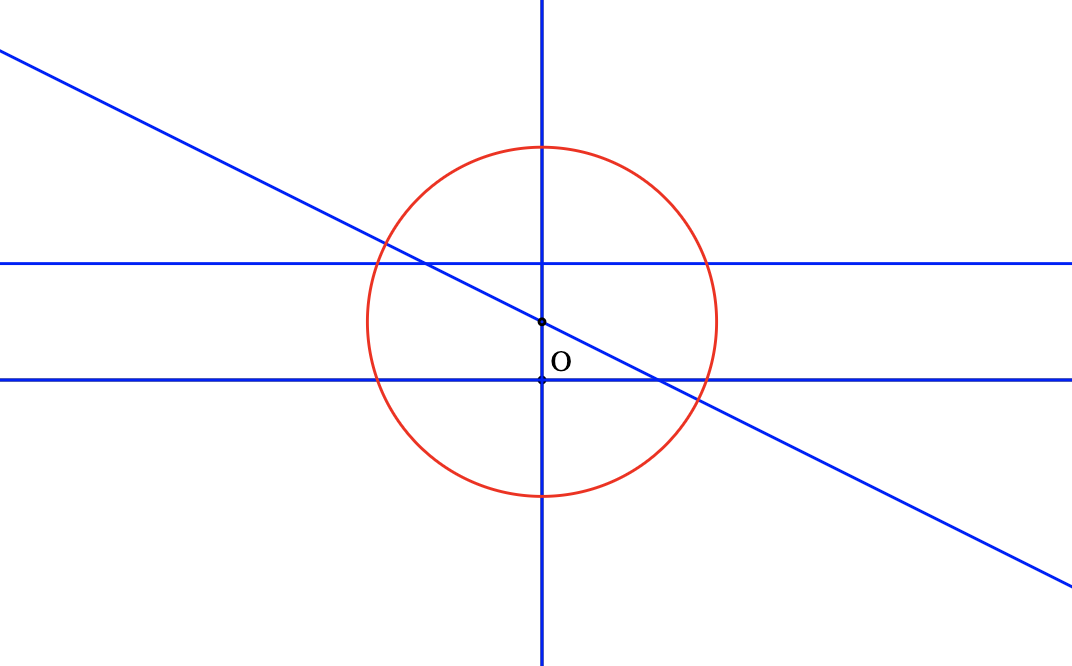시간 제한메모리 제한제출정답맞힌 사람정답 비율
20 초 (추가 시간 없음) 256 MB221100.000%

## 문제

Mr.Panda is playing a game named Watcher of Samsara, a famous tower defense game developed by a Chinese studio. At the beginning of the game, every player is able to choose their first hero arbitrarily from the hero pool which is randomly generated by the game system. Among all the heroes, Mr.Panda always chooses the hero named Leshrac, because of the character's ultimate skill, Pulse Nova.

Pulse Nova creates waves of damaging energy around Leshrac, one per second, to damage all nearby enemy units. This powerful skill inspires Mr.Panda to come up with a geometry problem.

There are $n$ straight lines on the 2D plane, where the $i^{th}$ line passes two integer points $P_i$ and $Q_i$. You need to place a circle with radius of exactly $R$ on the plane. For each given line, there might be a segment part of this line that will be inside the circle. Please find a position for this circle to maximize the total length of all segment parts which will be inside the circle.

## 입력

The first line of the input gives the number of test cases, $T$ $(1 \le T \le 100)$. $T$ test cases follow.

The first line of each test case contains two integers $n$ $(1 \le n \le 50)$ and $R$ $(1 \le R \le 3000)$, the number of straight lines, and the radius of the circle, respectively.

In the next $n$ lines, each contains four integers. In the $i^{th}$ line, the first two integers indicate the 2D coordinates of point $P_i$, and the last two integers indicate the 2D coordinate of point $Q_i$. We ensure that $P_i \ne Q_i$ and the absolute value of every coordinate is not greater than $1000$.

We ensure the sum of $n$ in all test cases is not greater than $100$.

## 출력

For each test case, output one line containing "Case #x: y", where x is the test case number (starting from 1) and y is the maximum length which will be inside the circle. Your answer will be considered correct if it is within an absolute or relative error of $10^{-6}$ when compared with the correct answer.

## 예제 입력 1

3
2 2
1 1 1 2
1 1 2 1
4 3
0 0 0 1
2 0 0 1
0 0 1 0
0 2 1 2
5 4
1 3 -2 3
0 0 4 0
0 1 -1 2
-3 1 2 -1
1 3 2 -3


## 예제 출력 1

Case #1: 8.0000000000
Case #2: 23.3137084990
Case #3: 38.0402955628


## 노트

For the first sample, you can place the center of the circle at $(1, 1)$, so the total length inside the circle will be $8$.

For the second sample, you can place the center of the circle at $(0, 1)$, so the total length inside the circle will be $12 + 8\sqrt{2}$.